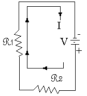#### Series Circuits Practice Problems

1. Given the series circuit below find the following unknown values.Show all your work step-by step

 GIVEN FIND FIND ITotal = 2 A VTotal= ? RTotal = ? V1 = 2 V R 1 = ? V2 = R2 = 10 Î© I1 = ? I2 = ?

2. Calculate the total power used by the above circuit

3. Add a third resistor in series to the above circuit.

The value of this resistor is R3 = 10 ohms.

The value of the current stays the same (ITotal = 2 A).

Recalculate all the values in the table above and the value of V3 and I3.